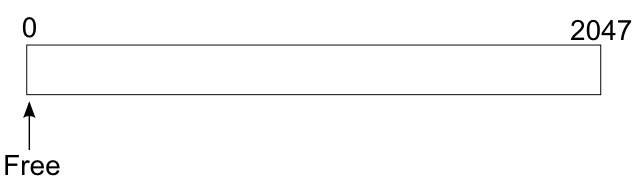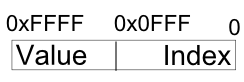# Introduction

This application is the attempt to resolve an exercise that was posted online by a well known game studio.

It is by no means the perfect solution. I think that it works the way it was intended for the challenge, but since I did not apply for the job, I cannot garantee it is the desired one.

# Challenge

The original challenge is as follows:

• To write a set of functions for managing a variable number of byte queues
• Each queue can have a variable length
• It is assumed the amount of available memory is small and fixed

The interface for the required function set is defined as:

``````Q * create_queue(); //Creates a FIFO byte queue, returning a handle to it.
void destroy_queue(Q * q); //Destroy an earlier created byte queue.
void enqueue_byte(Q * q, unsigned char b); //Adds a new byte to a queue.
unsigned char dequeue_byte(Q * q); //Pops the next byte off the FIFO queue.
``````

The execution of the following set of calls:

``````Q * q0 = create_queue();
enqueue_byte(q0, 0);
enqueue_byte(q0, 1);
Q * q1 = create_queue();
enqueue_byte(q1, 3);
enqueue_byte(q0, 2);
enqueue_byte(q1, 4);
printf("%d ", dequeue_byte(q0));
printf("%d\n", dequeue_byte(q0));
enqueue_byte(q0, 5);
enqueue_byte(q1, 6);
printf("%d ", dequeue_byte(q0));
printf("%d\n", dequeue_byte(q0));
destroy_queue(q0);
printf("%d ", dequeue_byte(q1));
printf("%d ", dequeue_byte(q1));
printf("%d\n", dequeue_byte(q1));
destroy_queue(q1);
``````

should give as output:

``````0 1
2 5
3 4 6
``````

The type Q presented in the interface description can be defined in any way the implementation requires.

The code is not allowed to call malloc() or other heap management routines. Instead, all storage (other than local variables in the functions) must be within a provided array:

``````unsigned char data;
``````

Memory efficiency is important. On average while the system is running, there will be about 15 queues with an average of 80 or so bytes in each queue.

The functions may be asked to create a larger number of queues with less bytes in each, or to create a smaller number of queues with more bytes in each.

Execution speed is important. Worst-case performance when adding and removing bytes is more important than average-case performance.

If a request cannot be satisfied due to lack of memory, the code should call a provided failure function, which will not return to the caller:

``````void on_out_of_memory();
``````

Similarly if the caller makes an illegal request, like attempting to dequeue a byte from an empty queue, a provided failure function should be invoked, which will not return back:

``````void on_illegal_operation();
``````

There may be spikes in the number of queues allocated, or in the size of an individual queue.

The code should not assume a maximum number of bytes in a queue (other than that imposed by the total amount of memory available, of course!). It can be assumed that no more than 64 queues will ever be created at once.

# Solution Overview

The solution is implemented in C++ (queue.cpp).

Given the amount of available memory (2048 bytes), and assuming that an int takes 4 bytes, we can use an int cell for storing the value and the pointer for the next queue element.

From the API list, we are only storing byte values, which leaves us with 3 bytes for the pointer part. This allows us to index up to 4096 bytes, which is much more than the 2048 bytes we have available. On the other hand we are able to manipulate the queue using a word size, which is register and memory bus friendly, hence fulfilling the memory efficiency and execution speed requirements, as shown in figure 2.

The solution is thus to start by having a pointer to the initial position of the memory and assume the complete memory storage is available, as shown in the figure 1.Figure 1, the initial state of the raw memory used to store the queues.Figure 2, the contents of a cell element when it has a value.

When a new element is allocated, the free pointer advances 4 bytes and the cell gets assigned the desired value. In case the element is being assigned to a queue, which has already some elements, the last element gets ajusted to point to the new cell as expected.

In the case the queue is being allocated, a small optimization is made, where the next element index has the value 0xFFF, which is invalid in our case (much bigger than 4096), this way the enqueue_byte() knows it does not to allocate a new cell on the first value.The next figure shows how the memory looks like after a few allocations.